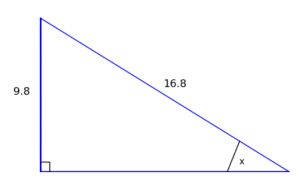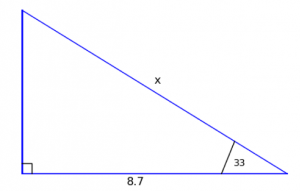My dad tells me that, above the blackboard in his 1960s Scottish high school, was a banner with the letters ‘SOH CAH TOA’ written out on it. Any questions about the banner were brushed off with a smile and ‘you’re not old enough to learn about SOH CAH TOA yet.”

Which, I have to concede, is a great way to pique kids’ interest in the topic. I’ve often wondered about the idea of telling students they’re not old enough to know about maths yet, it’s for over-16s only - and then let them get on with finding out the details on the sly.

### However, SOH CAH TOA is stupid - there, I said it

There are - if you count the SOH CAH TOA way - 11 types of right-angled triangle questions. (Finding the hypotenuse or a leg ((a short side’)) given the other two sides; three versions of finding the angle given the other two sides; and six versions of finding one side given an angle and another side.) If you ask me - and I suggest you do - that’s silly.

I say there are only two kinds of right-angled triangle problem: finding an angle if you know all the sides and finding a side if you know all the angles and a side. And all you need to know in order to solve all of these things: Pythagoras and the sine rule.

### Pythagoras

Now, you know Pythagoras. The square on the hypotenuse is equal to the sum of the squares on the other two sides - or, if you prefer, $opp^2 + adj^2 = hyp^2$. (I prefer this to $a^2 + b^2 = c^2$ because it tells you which side is which.) That means:

•  if you know the two short sides, you square them, add them up and square root the answer to get the hypotenuse;
• if you know the hypotenuse and a leg, you square them, take them away (bigger minus smallest, of course) and square root the answer to get the other leg.

That’s straightforward. If you’re solving triangles the Table of Joy way, it usually makes sense to find the last side, just in case you need it.

### The other angle

Oh! If you have two angles of a triangle, it’s easy to find the third, isn’t it? Especially if one of them happens to be a right angle. You simply work out $\frac{\pi}{2}$ minus the other angle.

What’s that?

Oh, fine. If you MUST use degrees (radians are much better), it’s 90 minus the other angle.

### The Sine Rule

At GCSE, you get given the sine rule on the front of the paper. At A-level, nope, you have to remember it. It’s not exactly difficult, though:

$\\frac{a}{\\sin(A)} = \\frac{b}{\\sin(B)} = \\frac{c}{\\sin(C)}$

What you do once you have either all three sides (and the right angle, don’t forget) or all three angles (and a side), is label the corners of the triangle like the picture, with each angle (a big letter) opposite its corresponding little letter.

Also, write out the sine rule, and put a circle around all of the information you have, and a square around the thing you don’t know. If there’s a fraction with one of its numbers unshaped, that’s fine - just cross it out. Neatly.

### The Sine Rule with the Table of Joy

Here comes the clever bit! You can even do this without drawing the whole table - but if you’re curious, you can buy Basic Maths For Dummiesand/or Numeracy Tests For Dummiesto see exactly how the Table of Joy works.

Here’s what you do:

1. Rewrite your fractions with numbers in the appropriate places and a question mark in the missing space.
2. Find the number diagonally opposite the question mark and write it on the bottom of a big fraction.
3. Take the other two numbers and write them out on top of the fraction with a times between them.
4. Work out the fraction you’ve just written down.
5. If you were looking for a side, you’re done. Hooray.
6. If you’re after an angle, do $\sin^{-1}(Ans)$ and that’ll give you the answer.

### Example: finding an angleWith this one, we don’t really need the bottom (adjacent) side, but let’s find it anyway: $16.8^2 - 9.8^2 = 186.2$, so the bottom side is the square root of that - 13.64 units (to 2dp). The Table of Joy would have 9.8 and 16.8 on the top, and $\sin(x)$ next to $\sin(\frac{\pi}{2})$ on the bottom. You’d work out $9.8 \times \sin(\frac{\pi}{2}) \div 16.8 = 0.583$; since we want an angle, we do inverse sine of that using the answer button to get 0.623 radians (38.69°, if you must).

### Finding a sideThis one gives an angle in degrees, tut tut. We can find the other angle by working out 90° - 33° = 57° and then work out the Table of Joy: you’ve got 8.7 and x on top, and $\sin(57^\circ)$ next to $\sin(90^\circ)$ on the bottom. The sum is $8.7 \times \sin(90^\circ) \div \sin(57^\circ) = 10.37$ units (2dp)

So there you go. A simple, easy way to solve any right-angled triangle without having to mess around with tan and cosine.

### Why is this better?

Oh yeah, why is this a better approach than slavishly learning all 11 possible right-angled triangle types? Because this method also works for non-right-angled triangles - although you also need the cosine rule instead of Pythagoras for some of those. It seems daft to me to learn a dozen different ways of doing special cases when you can learn a handful of general cases and be done with it. So there!

(Image by fdecomite used under a Creative Commons by licence)

* Edited 16/12/2013 for formatting.